dolphins-part1

# Dolphin Society Networks in Doubtful Sound, NZ, part 1¶

When I saw this dolphin society network dataset, I thought it was a great way to merge some interests I have in Marine Biology and network algorithms. The data comes from Network Repository, which provides data in NIST mtx format for download. Even though I fancied myself pretty familiar with the many different flavors of graph notation, this was yet another one I had never seen before. Luckily some googling yielded a similar question in SageMath with regard to mtx format with an elegant solution, and it worked quite well to get this in a format suitable for manipulation with networkX.

In :
import pandas as pd

In :
%pylab inline

Populating the interactive namespace from numpy and matplotlib

In :
from scipy.io import mmread
import networkx as nx
import numpy as np

In :
!ls *mtx

soc-dolphins.mtx


To summarize the following one-liner from SageMath, the solution involved reading the file into a Scipy sparse matrix using mmread, then converting to a dense matrix, then converting the matrix type to numpy, then using networkX to create the graph object.

In :
g = nx.Graph(np.matrix(mmread('soc-dolphins.mtx').todense()))

In :
g

Out:
<networkx.classes.graph.Graph at 0x112c0c240>

# Basic networkX statistics and visualization¶

Number of nodes and edges are the two first network stats that come to mind. On the Network repository site, number of nodes is 62. Number of edges, 159. This should be the same.

In :
len(list(g.nodes))

Out:
62
In :
len(list(g.edges))

Out:
159

Our dataset has 62 nodes (dolphins) and 159 edges (relationships between dolphins) as the metadata from Network Repository said.

Unfortunately, we lack the labels for the data, but we can still calculate some simple network metrics.

In :
nx.average_node_connectivity(g)

Out:
3.0634584875727127

This is somewhat confusing, given that the average node connectivity, k was given as 4.97 in this paper but it could be that the original author has weighted edges that are not shown in the shared files or that the software he used calculates k differently.

In :
sum(list(nx.triangles(g).values()))/3

Out:
95.0

The number of triangles within the network is 95.

NetworkX has some built-in drawing functions, which we can use to get an idea of the data.

In :
nx.draw(g, with_labels=True, font_weight='bold', node_color="pink")

/Users/gbang/anaconda3/lib/python3.6/site-packages/networkx/drawing/nx_pylab.py:611: MatplotlibDeprecationWarning: isinstance(..., numbers.Number)
if cb.is_numlike(alpha):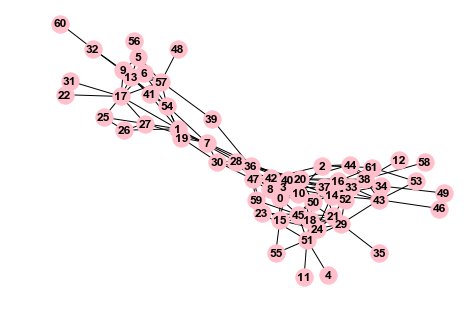In :
nx.draw_spring(g, with_labels=True, font_weight='bold', node_color="pink")

/Users/gbang/anaconda3/lib/python3.6/site-packages/networkx/drawing/nx_pylab.py:611: MatplotlibDeprecationWarning: isinstance(..., numbers.Number)
if cb.is_numlike(alpha):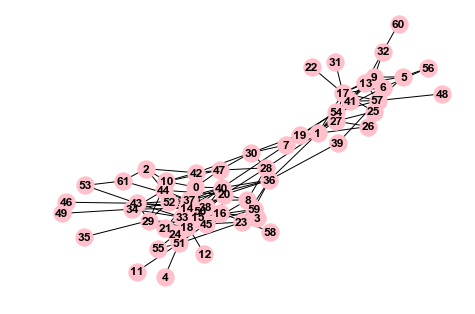In :
nx.draw_kamada_kawai(g, with_labels=True, font_weight='bold', node_color="pink")

/Users/gbang/anaconda3/lib/python3.6/site-packages/networkx/drawing/nx_pylab.py:611: MatplotlibDeprecationWarning: isinstance(..., numbers.Number)
if cb.is_numlike(alpha):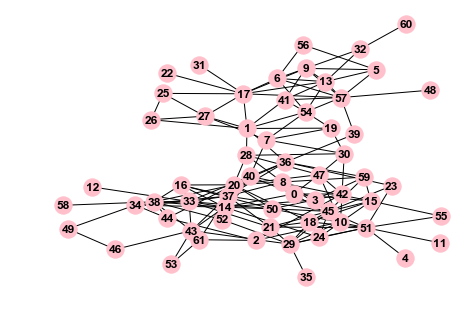In :
pos = nx.circular_layout(g)
nx.draw(g, pos, with_labels=True, node_color="pink")

/Users/gbang/anaconda3/lib/python3.6/site-packages/networkx/drawing/nx_pylab.py:611: MatplotlibDeprecationWarning: isinstance(..., numbers.Number)
if cb.is_numlike(alpha):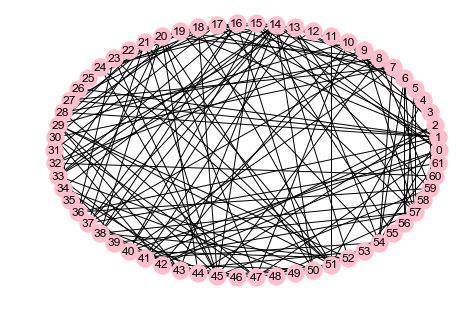There has definitely been prettier network output data, but this gives us a general idea of the beginning structure of the network. Graph visualization is a difficult problem, so using a tool dedicated to that task is recommended (e.g., not NetworkX). It might work to output NetworkX output to graphviz as well. As recommended in the NetworkX docs, we also recommend writing the graph output to GraphML (GML) which as they mention is compatible with Cytoscape and in my experience has been compatible with Gephi as well.

It is notable that some of these visualizations somewhat similar to the one shown in a later work by the same author, Lusseau:

In :
from IPython.display import Image

In :
Image("dolphinnetworkfig.png")

Out: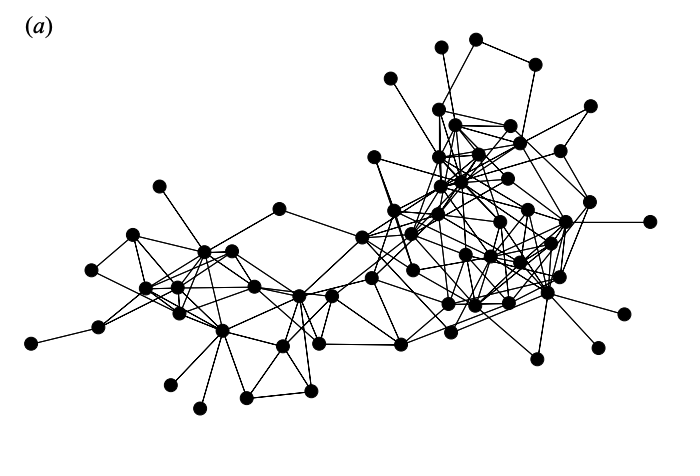We can use networkX write_gml function to write the graph to GML format.

In :
nx.write_gml(g, "dolphins.gml")

In :
!head dolphins.gml

graph [
node [
id 0
label "0"
]
node [
id 1
label "1"
]
node [


As a side note, I personally have quite enjoyed Gephi and mostly have learned it through the tutorials that have been made publicly available by the author, Clement Levallois, but I have also found that some tips and tricks have only been seen in the Gephi facebook group, so I would also recommend that new users to Gephi also join that community as well.

As another side note, this dolphins dataset has also been uploaded here by Dr. Mark Newman, in .gml format. A good homework assignment would be to check if these .gml files are exactly the same.

# Incidence of survey team¶

From the study (full text link) that these data were produced for, I learned that these dolphins live in a fjord, which is about as cold of a climate as it gets for bottlenose dolphins. The scientists conducted boat surveys using a 4.5-m vessel with a 50 hp engine from November 1994 to November 2001, so seven years' time, with 594 days (3284 hours) spent surveying during that time. Apparently, something happened in 1998 because that year no samples were taken. In any case, the survey route was the same each time (according to the researchers) and humans on the survey vessel would observe dolphins, whether they were in the same school (using visual distance perceived the human) and photo-identified (by the human) using dorsal fin markings. Big props to the researchers who painstakingly recorded this data while on a 4.5m boat or after the fact somehow.

If I could get the actual observation data, it would be interesting to do an analysis from the dolphins' point of view using survivorship statistics, considering this data left-censored -- how long could a dolphin relationship exist before this survey group discovered it? But since we don't have dates, we could just work out how many hours were observed out of all available in a given year using pandas datetimes and timedeltas and that should give us a decent idea of how much the survey team was out there.

In :
import pandas as pd

In :
tt={}
tt=pd.Timedelta(pd.to_datetime("1/1/1995 00:00:00PM")-
pd.to_datetime("11/1/1994 00:00AM"))
tt=pd.Timedelta(pd.to_datetime("1/1/1998 00:00AM")-
pd.to_datetime("1/1/1998 00:00AM"))
tt=pd.Timedelta(pd.to_datetime("11/1/2001 00:00AM")-
pd.to_datetime("1/1/2001 00:00:00PM"))

for year in [1995, 1996, 1997, 1999, 2000]:
tt[year]=pd.Timedelta(pd.to_datetime("12/31/"+str(year)+" 11:59:59PM")
-pd.to_datetime("1/1/"+str(year)+" 00:00AM"))

In :
tt

Out:
{1994: Timedelta('61 days 12:00:00'),
1998: Timedelta('0 days 00:00:00'),
2001: Timedelta('303 days 12:00:00'),
1995: Timedelta('364 days 23:59:59'),
1996: Timedelta('365 days 23:59:59'),
1997: Timedelta('364 days 23:59:59'),
1999: Timedelta('364 days 23:59:59'),
2000: Timedelta('365 days 23:59:59')}
In :
tt_hours={i:(tt[i]/np.timedelta64(1, 'h')) for (i,k) in tt.items()}

In :
tt_hours

Out:
{1994: 1476.0,
1998: 0.0,
2001: 7284.0,
1995: 8759.999722222223,
1996: 8783.999722222223,
1997: 8759.999722222223,
1999: 8759.999722222223,
2000: 8783.999722222223}

The study gave us a handy table denoting how many days/ hours were spent surveying each year, as well as the time (in hours) spent with dolphins.

In :
surveydata=pd.read_csv("dolphins_survey_team.txt",sep=" ", index_col=0)

In :
surveydata

Out:
Days_field Hours_field Hours_dolphins
Year
1995 133 621 418
1996 94 414 288
1997 78 415 256
1998 0 0 0
1999 107 759 533
2000 78 489 360
2001 56 319 275

To this we add a column giving the pandas TimeDeltas for the possible survey time each year.

In :
hours_pos_yr=pd.DataFrame(pd.Series(tt_hours), columns=["tot_hrs"])

In :
hours_pos_yr

Out:
tot_hrs
1994 1476.000000
1998 0.000000
2001 7284.000000
1995 8759.999722
1996 8783.999722
1997 8759.999722
1999 8759.999722
2000 8783.999722
In :
surveydata=pd.concat([surveydata,hours_pos_yr], axis=1)

In :
surveydata

Out:
Days_field Hours_field Hours_dolphins tot_hrs
1994 NaN NaN NaN 1476.000000
1995 133.0 621.0 418.0 8759.999722
1996 94.0 414.0 288.0 8783.999722
1997 78.0 415.0 256.0 8759.999722
1998 0.0 0.0 0.0 0.000000
1999 107.0 759.0 533.0 8759.999722
2000 78.0 489.0 360.0 8783.999722
2001 56.0 319.0 275.0 7284.000000

Apparently data from 1994 are not given, so we calculated tot_hrs for 1994 for nothing. We can just drop that year and now calculate the dolphin hours per total hour on the field and per possible hour.

In :
surveydata=surveydata.dropna()


In a calculation that the author has doubtlessly performed before, the hours_dolphins divided by hours_field are given below:

In :
surveydata["Hours_dolphins"]/surveydata["Hours_field"]

Out:
1995    0.673108
1996    0.695652
1997    0.616867
1998         NaN
1999    0.702240
2000    0.736196
2001    0.862069
dtype: float64

This is definitely a pretty high rate, especially on a per-hour basis, so there must be tons of dolphins in Doubtful Bay that are being observed by this group if in 2001, 86% of hours spent in the field involved at least one dolphin sighting (if I'm understanding the methods section correctly).

In :
surveydata["dolphinfieldperc"]=surveydata["Hours_dolphins"]/surveydata["Hours_field"]

/Users/gbang/anaconda3/lib/python3.6/site-packages/ipykernel_launcher.py:1: SettingWithCopyWarning:
A value is trying to be set on a copy of a slice from a DataFrame.
Try using .loc[row_indexer,col_indexer] = value instead

See the caveats in the documentation: http://pandas.pydata.org/pandas-docs/stable/indexing.html#indexing-view-versus-copy
"""Entry point for launching an IPython kernel.

In :
import seaborn as sns

In :
sns.set_style("whitegrid")

In :
surveydata["dolphinfieldperc"].plot.line()

Out:
<matplotlib.axes._subplots.AxesSubplot at 0x1a2712ee80>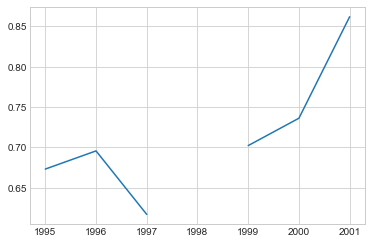With somewhat of a dip in 1997 (impending end of funding?), the rate of dolphin sighting in an hour of fieldwork generally increased over the years of the study. Many possible explanations for this, but likely the increase in ability of the graduate student in performing the photo-ID is a large factor in this.

In :
surveydata["Hours_field"]/surveydata["tot_hrs"]

Out:
1995    0.070890
1996    0.047131
1997    0.047374
1998         NaN
1999    0.086644
2000    0.055669
2001    0.043795
dtype: float64

It seems that hours on the field/year peaked in year 5 of the PhD. What if we just reduced the set of tot_hrs in a year to just the daylight hours in a year? I used the sun.py code from the second best answer in this stackoverflow link but changed a line or two to make the function take the particular day in question as arguments (given in day, month, year) instead of the current day. The version with those tiny changes is in this gist.

Anything that calculates a sunrise and sunset time requires a latitude and longitude, so a quick google search reveals that Doubtful Sound is located at 45° 19' 21.8172'' S and 166° 59' 32.4780'' E. That's pretty close to longitude 167 and latitude -45.3 in the terms that this Sun class requires.

In :
from Sun import Sun

coords = {'longitude' : 167.0, 'latitude' : -45.3 }

sun = Sun()

# Sunrise time UTC (decimal, 24 hour format)
print(sun.getSunriseTime( coords , day=1, month=1, year=2001)["decimal"])

# Sunset time UTC (decimal, 24 hour format)
print(sun.getSunsetTime( coords, day=1, month=1, year=2001)["decimal"])

17.129900718899165
8.711706795497829


Here we get the difference between the numbers and we now know that on January 1st, 2001 in Doubtful Bay NZ there were around 8.42 hours of daylight to enjoy.

In :
sun.getSunriseTime( coords , day=1, month=1, year=2001)["decimal"]-(sun.getSunsetTime( coords, day=1, month=1, year=2001)["decimal"])

Out:
8.418193923401336

If we do this for all of the days in 2001, we can get the total hours of daylight in 2001.

In :
from datetime import timedelta, date

def daterange(date1, date2):
for n in range(int ((date2 - date1).days)+1):
yield date1 + timedelta(n)

start_dt = date(2001, 1, 1)
end_dt = date(2001, 11, 1)

def tot_dlhrs(date1, date2):
tot_dl=0
for i in daterange(date1, date2):
daylight=sun.getSunriseTime( coords , day=i.day, month=i.month,
year=i.year)["decimal"]-sun.getSunsetTime( coords, day=i.day, month=i.month, year=i.year)["decimal"]
tot_dl=tot_dl+daylight

In :
tot_dlhrs(date(2001, 1, 1), date(2001, 11, 1))

Out:
3812.0323779984046

Out of all daylight hours, what portion of those were field hours where the researcher would have a chance at observing a dolphin? Of course we can't expect this to be too high especially as we have not factored in weather at all.

We now calculate the number of total daylight hours available in the time range of each year of the study.

In :
td={}
td=tot_dlhrs(pd.to_datetime("11/1/1994 00:00AM"),pd.to_datetime("1/1/1995 00:00:00AM"))
td=tot_dlhrs(pd.to_datetime("1/1/2001 00:00:00PM"),pd.to_datetime("11/1/2001 00:00AM"))

for year in [1995, 1996, 1997, 1998, 1999, 2000]:
td[year]=tot_dlhrs(pd.to_datetime("1/1/"+str(year)+" 0:0:00AM"),pd.to_datetime("1/1/"+str(year+1)+" 00:00AM"))

In :
td

Out:
{1994: 546.3240453888795,
2001: 3802.179397990956,
1995: 4348.503443379837,
1996: 4356.917999081977,
1997: 4348.503443379837,
1998: 4348.503443379837,
1999: 4348.503443379837,
2000: 4356.917999081977}
In :
pd.DataFrame(pd.Series(td), columns=["tot_dl_hrs"])

Out:
tot_dl_hrs
1994 546.324045
2001 3802.179398
1995 4348.503443
1996 4356.917999
1997 4348.503443
1998 4348.503443
1999 4348.503443
2000 4356.917999
In [ ]:


In :
surveydata

Out:
Days_field Hours_field Hours_dolphins tot_hrs dolphinfieldperc tot_hrs
1994 NaN NaN NaN NaN NaN 1476.000000
1995 133.0 621.0 418.0 8759.999722 0.673108 8759.999722
1996 94.0 414.0 288.0 8783.999722 0.695652 8783.999722
1997 78.0 415.0 256.0 8759.999722 0.616867 8759.999722
1998 0.0 0.0 0.0 0.000000 NaN 0.000000
1999 107.0 759.0 533.0 8759.999722 0.702240 8759.999722
2000 78.0 489.0 360.0 8783.999722 0.736196 8783.999722
2001 56.0 319.0 275.0 7284.000000 0.862069 7284.000000
In :
surveydata=pd.concat([surveydata,pd.DataFrame(pd.Series(td), columns=["tot_dl_hrs"])], axis=1)

In :
surveydata

Out:
Days_field Hours_field Hours_dolphins tot_hrs dolphinfieldperc tot_hrs tot_dl_hrs
1994 NaN NaN NaN NaN NaN 1476.000000 546.324045
1995 133.0 621.0 418.0 8759.999722 0.673108 8759.999722 4348.503443
1996 94.0 414.0 288.0 8783.999722 0.695652 8783.999722 4356.917999
1997 78.0 415.0 256.0 8759.999722 0.616867 8759.999722 4348.503443
1998 0.0 0.0 0.0 0.000000 NaN 0.000000 4348.503443
1999 107.0 759.0 533.0 8759.999722 0.702240 8759.999722 4348.503443
2000 78.0 489.0 360.0 8783.999722 0.736196 8783.999722 4356.917999
2001 56.0 319.0 275.0 7284.000000 0.862069 7284.000000 3802.179398
In :
surveydata["Hours_field"]/surveydata["tot_dl_hrs"]

Out:
1994         NaN
1995    0.142808
1996    0.095021
1997    0.095435
1998    0.000000
1999    0.174543
2000    0.112235
2001    0.083899
dtype: float64

It seems that 1999 and 1995 were the most productive years, at least in terms of hours on the field. Another expansion to the problem would be to see whether this was due to weather or El Nino patterns or some other human-related reasons. But we now generally know what percentage of daylight hours were used for observation, and if we could have a record of what dolphins were observed at which time (the original data) we could use a Kaplan-Meier plot to measure the incidence of dolphin relationship exposure to the researcher. Some food for thought.

In [ ]:



# Hierararchical clustering¶

Since we had the sparse matrix in the original data, we construct a distance matrix using SciPy.

In :
from scipy.spatial.distance import pdist

In :
np.matrix(mmread('soc-dolphins.mtx').todense())

Out:
matrix([[0., 0., 0., ..., 0., 0., 0.],
[0., 0., 0., ..., 0., 0., 0.],
[0., 0., 0., ..., 0., 0., 1.],
...,
[0., 0., 0., ..., 0., 0., 0.],
[0., 0., 0., ..., 0., 0., 0.],
[0., 0., 1., ..., 0., 0., 0.]])
In :
dolphins_spm=pdist(np.matrix(mmread('soc-dolphins.mtx').todense()))

In :
dolphins_spm.shape

Out:
(1891,)

From this, I can simply reuse the code from this entry.

In :
from matplotlib.colors import rgb2hex, colorConverter
from collections import defaultdict
class Clusters(dict):
def _repr_html_(self):
html = '<table style="border: 0;">'
for c in self:
hx = rgb2hex(colorConverter.to_rgb(c))
html += '<tr style="border: 0;">' \
'<td style="background-color: {0}; ' \
'border: 0;">' \
'<code style="background-color: {0};">'.format(hx)
html += c + '</code></td>'
html += '<td style="border: 0"><code>'
html += repr(self[c]) + '</code>'
html += '</td></tr>'

html += '</table>'

return html
def get_cluster_classes(den, label='ivl'):
cluster_idxs = defaultdict(list)
for c, pi in zip(den['color_list'], den['icoord']):
for leg in pi[1:3]:
i = (leg - 5.0) / 10.0
if abs(i - int(i)) < 1e-5:
cluster_idxs[c].append(int(i))

cluster_classes = Clusters()
for c, l in cluster_idxs.items():
#the following line was changed to make
#the labels the same as node labels
i_l = [den[label][i] for i in l]
cluster_classes[c] = i_l

return cluster_classes

def get_clust_graph(data_dist, numclust, transpose=False, dataname=None, save=False, xticksize=8):
#myInd = [i for i, c in zip(B['ivl'], B['color_list']) if c=='g']
get_cluster_classes(B)
ax=plt.gca()
ax.tick_params(axis='x', which='major', labelsize=xticksize)
ax.tick_params(axis='y', which='major', labelsize=15)
#plt.xlabel(xl)
#plt.set_size_inches(18.5, 10.5)
plt.ylabel('Distance')
plt.xlabel('Dolphin')
plt.suptitle("", fontweight='bold', fontsize=16);
#labels = [item.get_text() for item in ax.get_xticklabels()]
#ax.set_xticklabels(labels)
if save:
plt.savefig(str(df.index.name)+str(numclust)+"tr_"+str(transpose)+"dn_"+str(dataname)+save+'.png')
else:
print("Not saving")
return get_cluster_classes(B)

In :
from pylab import rcParams
rcParams['figure.figsize'] = 12, 9

In :
get_clust_graph(dolphins_spm,62,xticksize=11)

Not saving

Out:
 b ['14', '33', '37', '15', '51', '45', '17', '54', '38', '50', '29', '0', '30', '8', '59', '36', '40', '1', '20'] g ['9', '13', '57'] r ['21', '24', '18'] c ['10', '42', '47'] m ['43', '52', '16', '34'] y ['7', '19', '28', '5', '6', '41', '32', '25', '26', '27', '46', '53', '39', '48', '4', '11', '55', '22', '31', '12', '35', '58', '60', '49', '56', '23', '3', '61', '44', '2']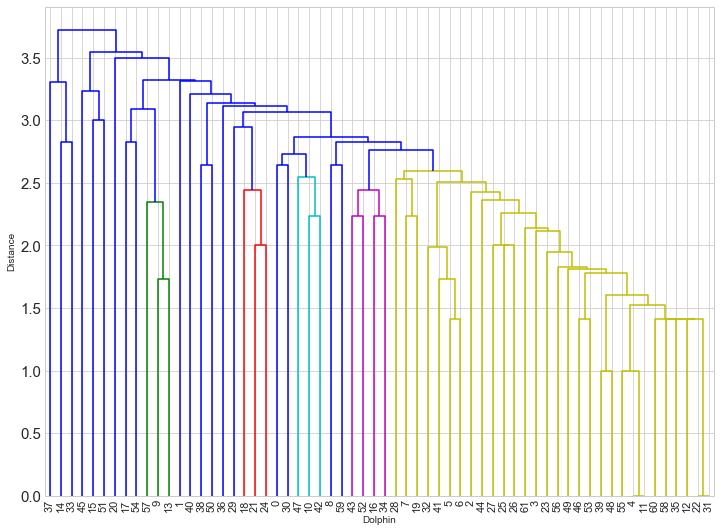Unfortunately, without the original HWI data (giving the actual distance between dolphins in relationship-space) given in the paper, it is impossible to recreate the dendrogram given in that paper exactly. However we can identify groups of dolphins that seem to have affinity.

Astute readers will note that the labels for the dolphins start at base 0 rather than 1 as in the original dataset. Therefore the dolphins are labeled 0-61, not 1-62.